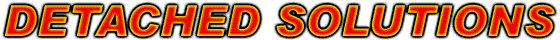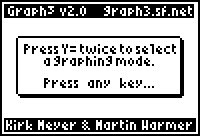www.detachedsolutions.comGraph3 v2.0
by Kirk Meyer & Martin Warmer

# Introduction

Graph3 adds a 3D grapher and a differential equation grapher to your TI-83+, TI-83+ Silver Edition, TI-84+ or TI-84+ Silver Edition. The 3D grapher is faster than the TI-89s built-in grapher.  It features rotation, zooming, tracing, and more.  It also draws either wireframe or hidden surface plots. The differential equation grapher features the Runge-Kutta method, slope fields and more.# Installation

Send the "graph3.8xk" file to your calculator using a graph link. You must have a link; applications cannot be typed in by hand.

To install, press [APPS] and then select "Graph3" from the list. A message box will popup, instructing you on how to proceed. (If you have other graphing applications installed, for example, Inequality or Transformation graphing, you will be asked to uninstall them.)

# Compatibility

Graph3 is not compatible with programs such as Inequality Graphing or Transformation Graphing. If you have these applications installed, Graph3 will request that you uninstall them in order to proceed. Graph3 may be installed at the same time as Symbolic and Omnicalc. However, for compatibility reasons, it does not allow you to enter their tokens into the Z= equation.

# Functionality

To enter the graphing modes, press [Y=] twice. A menu will popup where you can choose to enter Differential Equation or 3D graphing mode. You will remain in the selected mode until you push a key that exits graph mode (examples: [2nd]QUIT, program editor, memory check, etc). Graphing is very similar to the normal function graphing on the 83+, with the following differences:

### 3D Mode

• There are only two graph styles: wireframe (thin line) and hidden surface (filled triangle).
• [X,T,theta,n] inserts X as usual, but [STAT] inserts Y.
• Only three zooming options are available. Also, the cursor for zoom in and zoom out point selection is the same as the trace cursor to increase visibility.
• The Format menu has less options. Also, selecting PolarGC in 3D mode is the equivalent of a cylindrical coordinate system.
• [2nd]Stat Plot, [2nd]TblSet, [2nd]Calc, and [2nd]Table are not available in 3D mode.

There are also the following additional features:

• When viewing a graph (no cursor), push [GRAPH] again to instantly toggle the graph type between wireframe and hidden surface.
• Whenever viewing a graph, press [ALPHA]X, [ALPHA]Y, or [ALPHA]Z to view the graph from that axis.

### Differential Equation mode

#### Examples

To graph the differential equation y'(t)=2t with initial condition y(1)=0 we enter the following:

1. Go to the Y= screen
2. Enter in Y1' the equation "2T"
3. Enter in Yi1 the initial Y-value "0"
4. Go to the Window screen
5. Enter in Tmin the initial t-value "1"
6. Press the graph button

To graph the differential equation y''(t)=-y' with initial conditions y(0)=1 and y'(0)=0 we have to do the following:

1. Rewrite the equation as the system of equations y1'=y2 and y2'=-y1 (You may check that y1 is the same as the y function above.)
2. Go to the Y= screen
3. Enter in Y1' the equation "Y2" (Vars, Y-Vars, Y2)
4. Enter in Yi1 the initial Y-value "1"
5. Enter in Y2' the equation "-Y1" (Vars, Y-Vars, Y1)
6. Enter in Yi2 the initial Y-value "0"
7. Go to the Window screen
8. Enter in Tmin the initial t-value "0"
9. Press the graph button

#### Features

The Y= screen works as follows (for Y1 through Y6):
• Y1': The equation to be graphed
• Yi1: The initial Y-value for the equation.
The window screen has the following variables:
• Tmin: Sets the initial value of the indepent variable t. This is also the minimum value of t which will be graphed.
• Tmax: Sets the maximum value of the indepent variable t that will be graphed.
• Tstep: Sets the stepsize of the indepent variable t.
• Xmin/Xmax/Xscl: Sets the visible part of the X-axis.
• Ymin/Ymax/Yscl: Sets the visible part of the Y-axis.
• Diftol: Sets the tolerance for the RungeKutta method, which is used to select a stepsize for the RungeKutta algorithm. This variable is only visible when RungeKutta mode is selected.
• Fldres: Sets the number of columns (1 to 48) that are used to draw a slopefield. This value is only visible when the slopefield is enabled.
The format screen has the following new options:
• Euler/RungeKutta: Selects the solution method of the differential equation. You can choose between the faster Euler method or the more precise RungeKutta method.
• Field: Controls whether a slopefield of the equation is displayed. When the slopefield is on, graphing multiple equations or a system of equations will give an ERR:INVALID error.

# Uninstallation

To uninstall, simply run the application again (from the [APPS] menu). It should display a message indicating that it has been installed.

 IMPORTANT: Some applications will offer to uninstall Graph3. This will probably work, but it would be better to uninstall it yourself.

# Version History

 v2.0 Actual release containing differential equation support. v1.9 (beta) Differential Equation support added by Martin Warmer, done as a project for the Google Summer of Code. License changed to MIT License. v1.1 The application's name has been changed to "Graph3" to fix compatibility problems with the TI-84. Source code is also included with this version. v1.0 Many bugs fixed, including compatibility problems with OS version 1.13. Tracing problem also fixed. Added a few minor features and another easter egg (yes, there are two now). v0.9 Initial release.

# Known Issues

### 3D Mode

• Cannot use [2nd]Calc to enter abstract coordinates in trace mode
• [2nd]Left and [2nd]Right do nothing when viewing/tracing the graph

### Differential Equation Mode

• Recalling Y1 inserts Real(34,0 in the equation instead of the contents of Y1'.
• When entering Y1(3) for example, the (3) part is ignored when graphing, but used when calculating the table. This can cause the calculator to never finish calculating the table or display different results for the table that are different from the graph.
• Entering X in an equation will give an error when graphing, but when calculating the table it won't give an error; instead it will behave like you entered T.

# Thank You's

Thanks go out to...

• Michael Vincent (incessant rambling exchange)
• Dan Englender (help with hooks, suggestions)
• Rob van Wijk (great tester; many bug reports and suggestions)
• Jonah Cohen (for making and maintaining cz80)
• Matt Shepcar (I wouldn't have thought fast 3D was possible until I saw his torus demo)
• Kevin Kofler (comments and suggestions on diffequ mode)

Other people who have made suggestions and reported bugs so far:

• Shane Fry
• Patrick Poon
• Medevo
• DarkSlasher117
• Charlemagne
• Benny van Zuiden